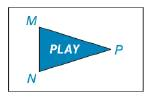Chapter 2.4, Problem 41E### Elementary Geometry for College St...

6th Edition
Daniel C. Alexander + 1 other
ISBN: 9781285195698

#### Solutions

Chapter
Section### Elementary Geometry for College St...

6th Edition
Daniel C. Alexander + 1 other
ISBN: 9781285195698
Textbook Problem
1 views

# The triangular symbol on the “PLAY” button of a DVD has congruent angles at M and N. If m ∠ P = 30 ∘ , what are the measures of angle M and angle N?To determine

The measures of angle M and angle N.

Explanation

Given:

The triangular symbol on the “PLAY” button has congruent angles at M and N.

mMmN and mP=30°.

Figure (1)

Property used:

The sum of the three angles in a triangle is 180°.

Definition:

If the two angles of a triangle is congruent, then the measures of the angles are same.

mM=mN

Calculation:

Calculate the angle M and angle N.

mP+mM+mN=180°(1)

Substitute 30° for mP in the equation (1)

### Still sussing out bartleby?

Check out a sample textbook solution.

See a sample solution

#### The Solution to Your Study Problems

Bartleby provides explanations to thousands of textbook problems written by our experts, many with advanced degrees!

Get Started